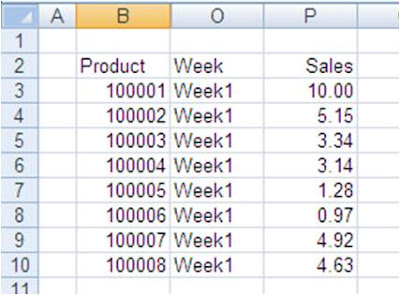## Sunday, November 22, 2009

### Excel Formula : Using Vlookup Function

One Golden Rule while working with Excel Formula's is never enter a hard coded value into Formula. If you can calculate a value with a formula, it should not be hard coded into your formula's

Today we will talk about how this is relevant in using Vlookup Function. We all use Vlookup function in our day to day working with the data in Excel. Given below is the Syntex for Vlookup function just for reference purpose.

VLOOKUP(lookup_value, table_array, col_index_num, [range_lookup])Picture on the above is typical data table used by us. Column C to N are hidden in this just to keep the table visible on screen. We need to get the sales value using vlookup formula. Most of the users (including me till some time back) hardcode col_index_num. Most common use of Vlookup Function for getting data from the table like above will be a formula like this.
=VLOOKUP(B13,B3:P10,15,0)

Here lookup_value is given at Cell B13, table_array is a Range B3:P10, col_index_num is 15 as it is 15th Column starting from Column B, range_lookup is 0 as we are looking for exact match.

In above formula the col_index_num is hard coded. Formula will give correct results till you do not insert or delete columns in between. Once you have done so , all your formulas will not update sales figure but reference some other value.

However with the following formula you can overcome this issue. You can calculate the number of columns with the help of Columns function of Excel.
=VLOOKUP(B13,\$B\$3:\$P\$10,COLUMNS(B3:P3),0)

COLUMNS(B3:P3) will be calculated as 15 and in case you insert any column between table_array your formula will change to VLOOKUP(B13,\$B\$3:\$Q\$10,COLUMNS(B3:Q3),0). Now columns function withing your formula will be calculated as 16 giving you correct value. This makes your Vlookup function dynamic.

Do refer to my earlier tip on Dynamic Range Names to make table_array also dynamic.

You are welcome to share your way of entering Vlookup function by way of comments to this post.

1.Never use VLOOKUP.
Use Match/Index

=Index(RngToPick,Match(WhatToMatch,WhereToMatch,MatchType)

2.